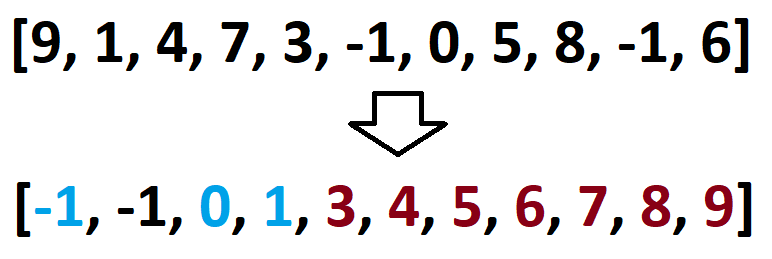# 【LeetCode】19 最长连续序列

## 题目

输入: [100, 4, 200, 1, 3, 2]



## 方法1 排序 思路## 方法1 排序 代码

package com.janeroad;

import java.util.Arrays;

public class test7 {
public int longestConsecutive(int[] nums) {
if (nums.length == 0) {
return 0;
}
Arrays.sort(nums);
int longestStreak = 1;
int currentStreak = 1;
for (int i = 1; i < nums.length; i++) {
if (nums[i] != nums[i-1]) {
if (nums[i] == nums[i-1]+1) {
currentStreak += 1;
}
else {
longestStreak = Math.max(longestStreak, currentStreak);
currentStreak = 1;
}
}
}
return Math.max(longestStreak, currentStreak);
}
public static void main(String[] args) {
int []num={2,4,3,-1,7,6,19,100};
test7 test7=new test7();
System.out.println(test7.longestConsecutive(num));
}
}


## 方法2 哈希表和线性空间的构造 代码

class Solution {
public int longestConsecutive(int[] nums) {
Set<Integer> num_set = new HashSet<Integer>();
for (int num : nums) {
}

int longestStreak = 0;

for (int num : num_set) {
if (!num_set.contains(num-1)) {
int currentNum = num;
int currentStreak = 1;

while (num_set.contains(currentNum+1)) {
currentNum += 1;
currentStreak += 1;
}

longestStreak = Math.max(longestStreak, currentStreak);
}
}

return longestStreak;
}
}